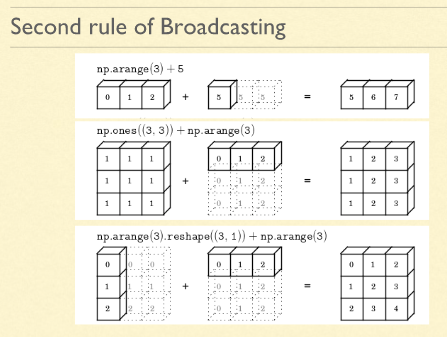# Numpy - Broadcasting in NumPy Arrays

In any NumPy mathematical operation, if the shape of any of the participating arrays is not sufficient, broadcasting rules come into play.

Broadcasting, generally, tries to adjust the shape of one or both of the arrays to bring them to required matching shape, by copying the existing elements required number of times, so that the mathematical operation could be performed between the two NumPy arrays.

First Rule: If the arrays do not have the same rank, then a 1 will be prepended to the smaller ranking arrays until their ranks match.

``````Q = np.array( [ [ [1, 3 ] ] ] )
P = np.array( [ 5 ] )

S = Q + P

print(S)
``````

This will print

``````[ [ [6, 8] ] ]
``````

Second Rule: Arrays with a 1 along with a particular dimension act as if they had the size of the array with the largest shape along that dimension. The value of the array element is repeated along that dimension.INSTRUCTIONS

(1) Please import the required libraries

``````import numpy as np
``````

(2) Please create a numpy array `X_broad` of shape (3,3) filled with ones

``````<<your code comes here>> = np.ones(<<your code comes here>>)
``````

(3) Print array `X_broad` using `print()` function to see its values

``````print(<<your code comes here>>)
``````

(4) Please create a numpy array `Y_broad` of size 3

``````Y_broad = np.arange(<<your code comes here>>)
``````

(5) Print array `Y_broad` using `print()` function to see its values

``````print(<<your code comes here>>)
``````

(6) Add `X_broad` and `Y_broad` and store the result in a variable `Z_broad`

``````<<your code comes here>> = <<your code comes here>>  + <<your code comes here>>
``````

(7) Print array `Z_broad` using `print()` function to see its values

``````print(<<your code comes here>>)
``````